Anúncio

# Leverage.pptx

25 de Sep de 2022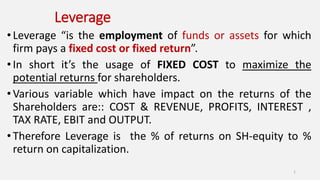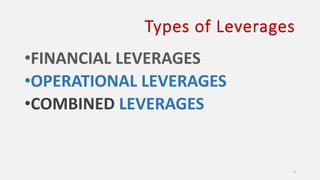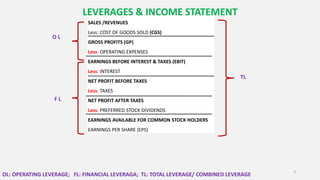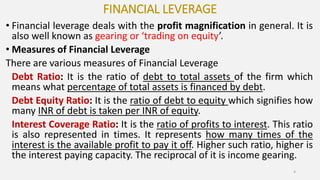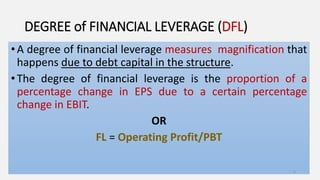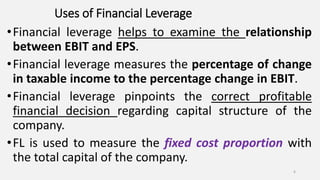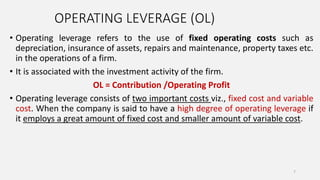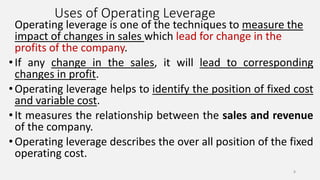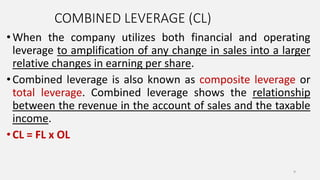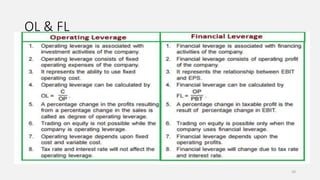1 de 10
Anúncio

### Leverage.pptx

1. Leverage • Leverage “is the employment of funds or assets for which firm pays a fixed cost or fixed return”. • In short it’s the usage of FIXED COST to maximize the potential returns for shareholders. •Various variable which have impact on the returns of the Shareholders are:: COST & REVENUE, PROFITS, INTEREST , TAX RATE, EBIT and OUTPUT. •Therefore Leverage is the % of returns on SH-equity to % return on capitalization. 1
2. Types of Leverages •FINANCIAL LEVERAGES •OPERATIONAL LEVERAGES •COMBINED LEVERAGES 2
3. SALES /REVENUES Less: COST OF GOODS SOLD (CGS) GROSS PROFITS (GP) Less: OPERATING EXPENSES EARNINGS BEFORE INTEREST & TAXES (EBIT) Less: INTEREST NET PROFIT BEFORE TAXES Less: TAXES NET PROFIT AFTER TAXES Less: PREFERRED STOCK DIVIDENDS EARNINGS AVAILABLE FOR COMMON STOCK HOLDERS EARNINGS PER SHARE (EPS) 3 O L F L TL OL: OPERATING LEVERAGE; FL: FINANCIAL LEVERAGA; TL: TOTAL LEVERAGE/ COMBINED LEVERAGE LEVERAGES & INCOME STATEMENT
4. FINANCIAL LEVERAGE • Financial leverage deals with the profit magnification in general. It is also well known as gearing or ‘trading on equity’. • Measures of Financial Leverage There are various measures of Financial Leverage Debt Ratio: It is the ratio of debt to total assets of the firm which means what percentage of total assets is financed by debt. Debt Equity Ratio: It is the ratio of debt to equity which signifies how many INR of debt is taken per INR of equity. Interest Coverage Ratio: It is the ratio of profits to interest. This ratio is also represented in times. It represents how many times of the interest is the available profit to pay it off. Higher such ratio, higher is the interest paying capacity. The reciprocal of it is income gearing. 4
5. DEGREE of FINANCIAL LEVERAGE (DFL) •A degree of financial leverage measures magnification that happens due to debt capital in the structure. •The degree of financial leverage is the proportion of a percentage change in EPS due to a certain percentage change in EBIT. OR FL = Operating Profit/PBT 5
6. Uses of Financial Leverage •Financial leverage helps to examine the relationship between EBIT and EPS. •Financial leverage measures the percentage of change in taxable income to the percentage change in EBIT. •Financial leverage pinpoints the correct profitable financial decision regarding capital structure of the company. •FL is used to measure the fixed cost proportion with the total capital of the company. 6
7. OPERATING LEVERAGE (OL) • Operating leverage refers to the use of fixed operating costs such as depreciation, insurance of assets, repairs and maintenance, property taxes etc. in the operations of a firm. • It is associated with the investment activity of the firm. OL = Contribution /Operating Profit • Operating leverage consists of two important costs viz., fixed cost and variable cost. When the company is said to have a high degree of operating leverage if it employs a great amount of fixed cost and smaller amount of variable cost. 7
8. Uses of Operating Leverage Operating leverage is one of the techniques to measure the impact of changes in sales which lead for change in the profits of the company. •If any change in the sales, it will lead to corresponding changes in profit. •Operating leverage helps to identify the position of fixed cost and variable cost. •It measures the relationship between the sales and revenue of the company. •Operating leverage describes the over all position of the fixed operating cost. 8
9. COMBINED LEVERAGE (CL) •When the company utilizes both financial and operating leverage to amplification of any change in sales into a larger relative changes in earning per share. •Combined leverage is also known as composite leverage or total leverage. Combined leverage shows the relationship between the revenue in the account of sales and the taxable income. •CL = FL x OL 9
10. OL & FL 10

### Notas do Editor

1. Eg: EBIT of the FIRM is Rs 50,000/-, Firms Tax bracket is 50%, Firm has EQ share capital of Rs 1,00,000/- ,Pref stock of Rs 1,00,000 @ 10% and Debentures valued at 1,25,000/ @ 8%. Calculate the firms Financial Leverage.
2. EBIT= Rs 50,000, Debt 1,25,000 @ 8%, Tax is 50%
3. FIRM- A & B Sales: Rs 25,00,000/- and Rs 30,00,000/- respectively; Fixed Costs: Rs 7,50,000/- & Rs 15,00,000/- respectively. Calculate Operating Leverage of Firm A & B, if Variable cost is 50% & 25% respectively of Sale for Firm A&B.
Anúncio